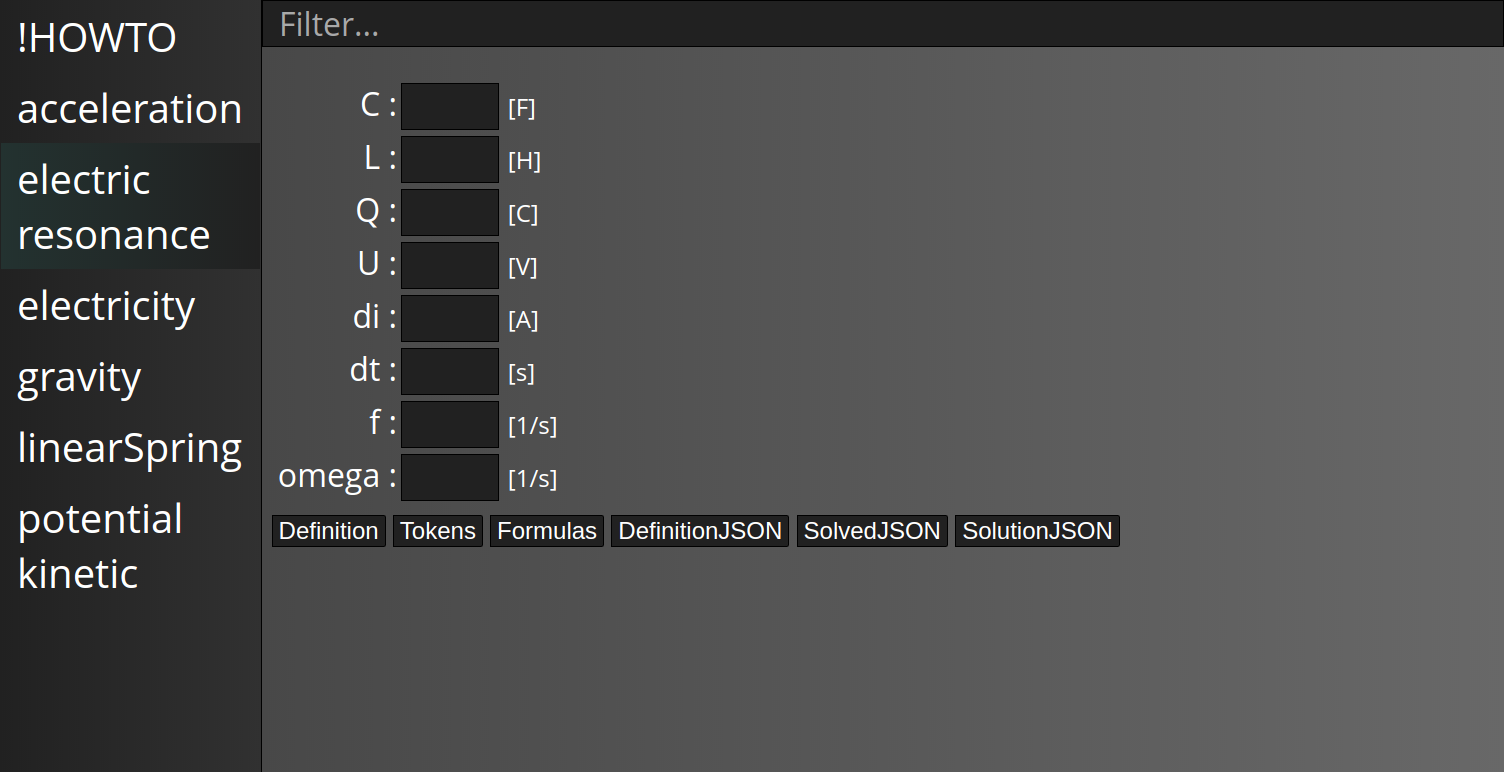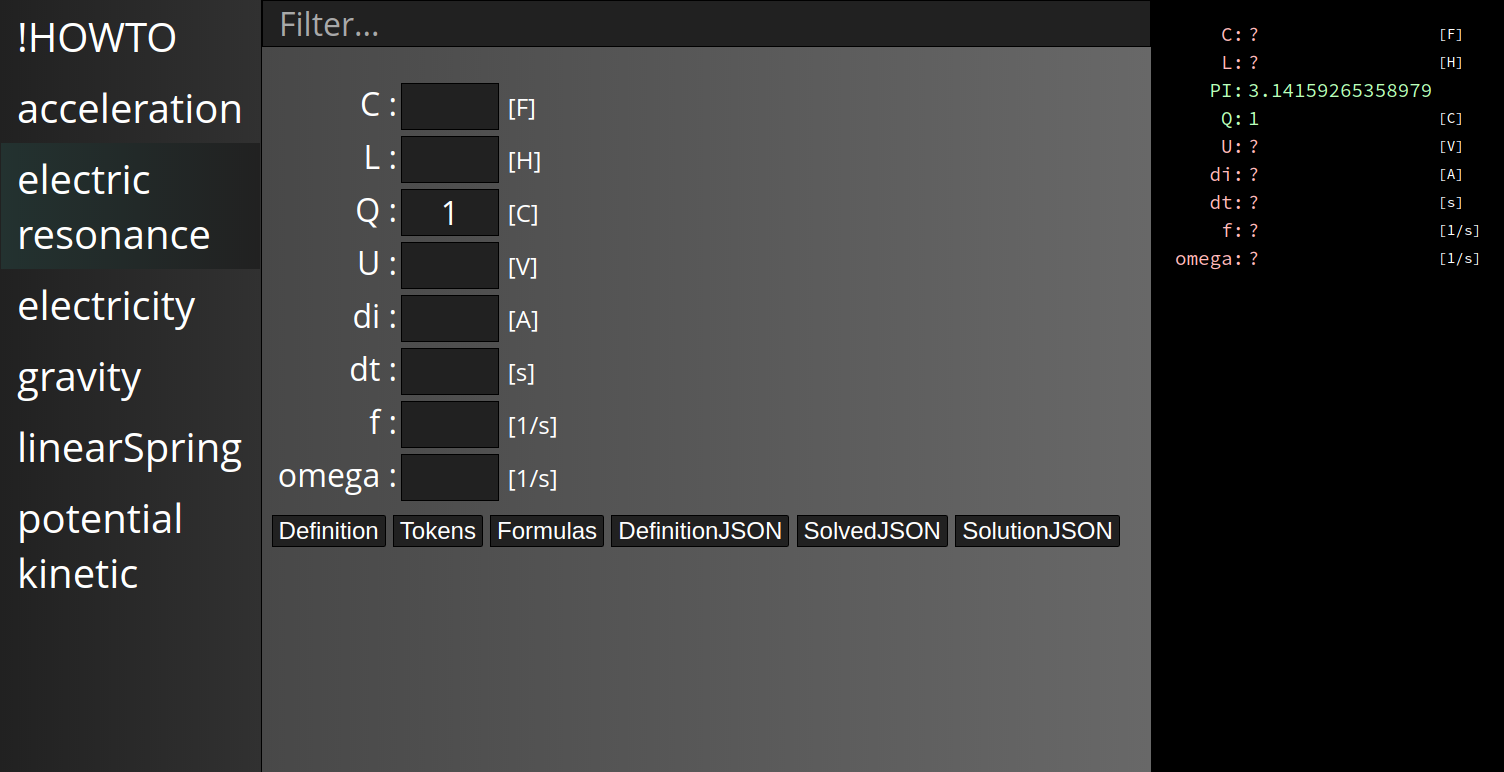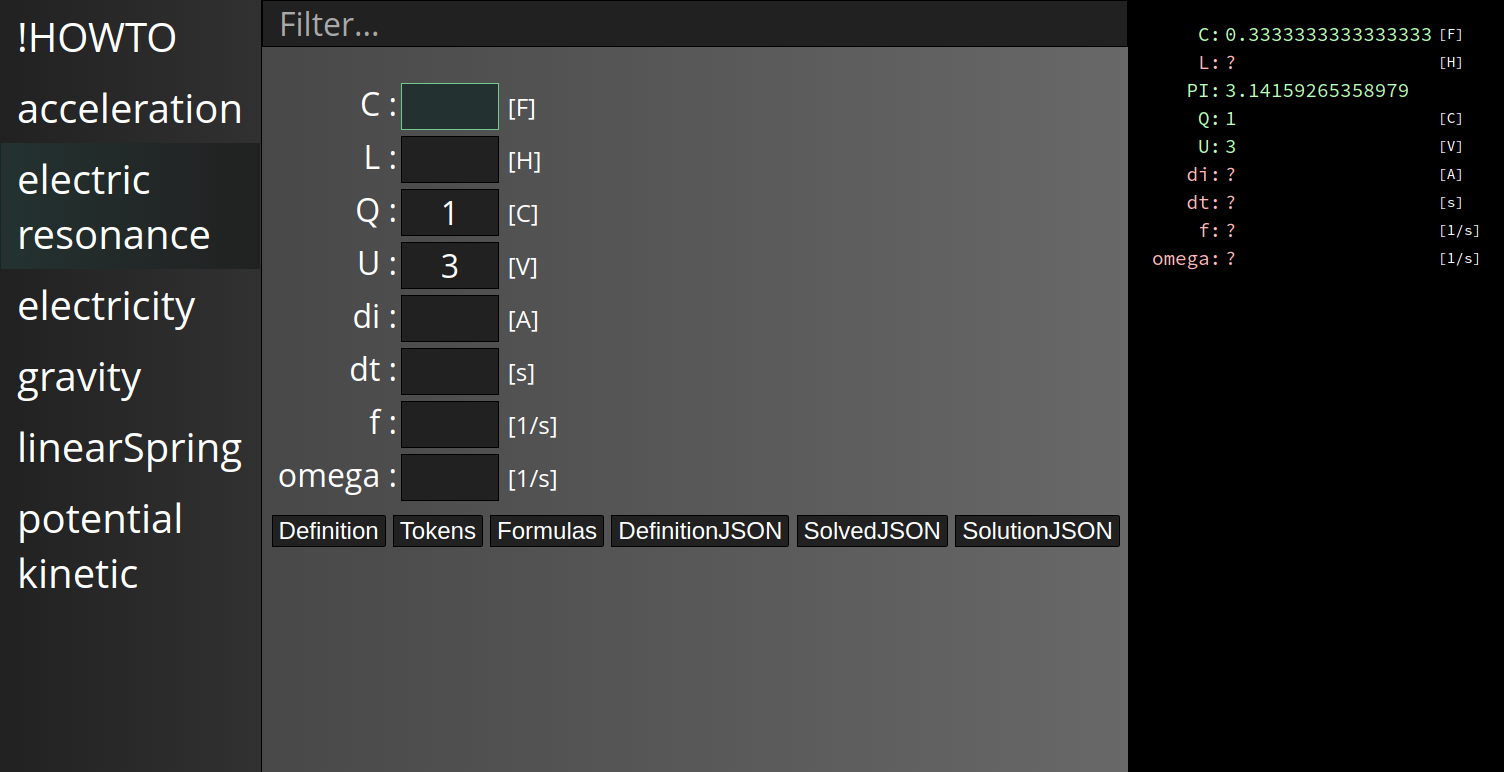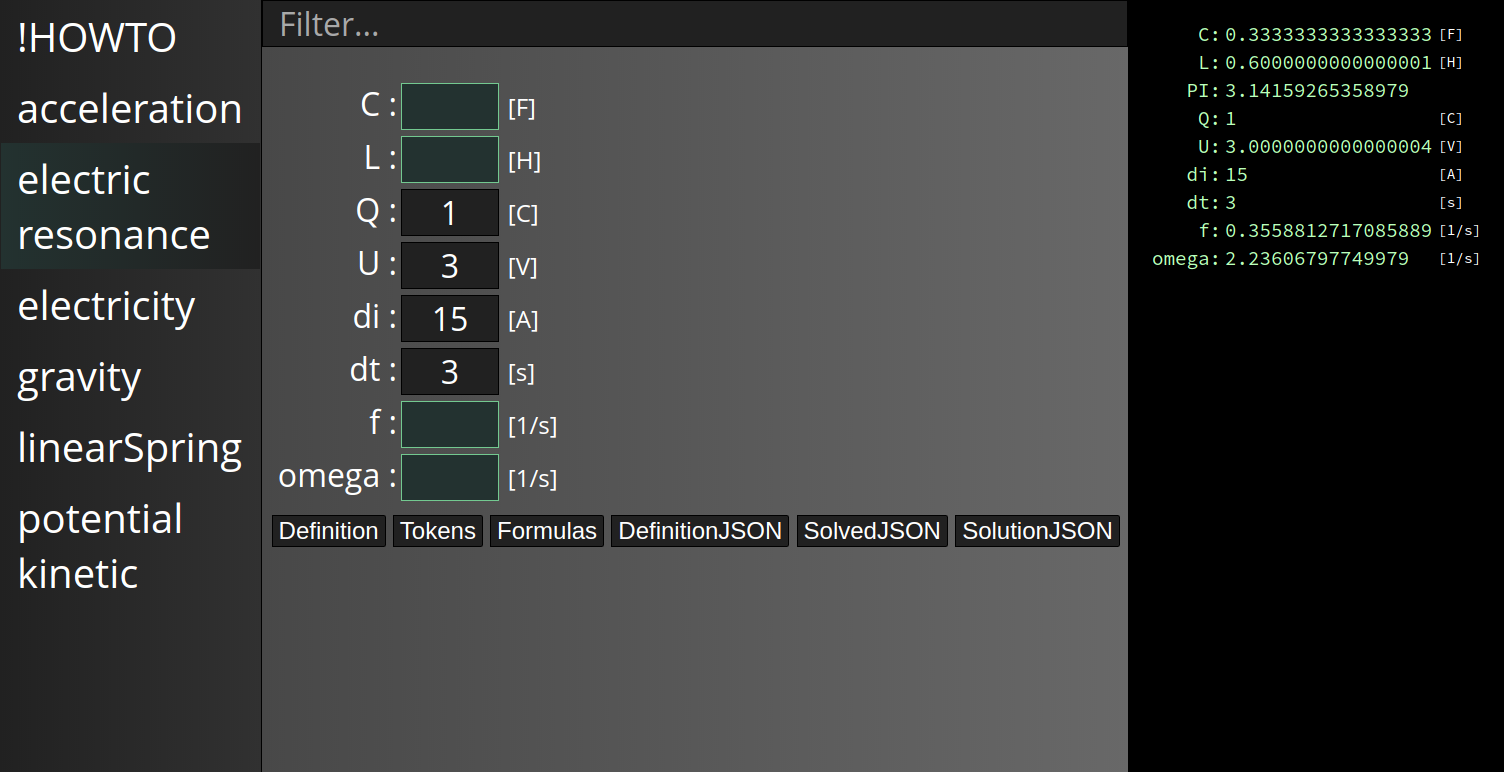# Solver

written in Haskell, Elm, HTML, CSS and JS

Currently closed source project I am working on.
The program can be used to solve any given set of equations by providing some / all of its input parameters. Which parameters are used as inputs or calculated by formulas can be decided by the `user`. Unlike with most other calculation software, there is no predefined `flow` of the calculation.
A variable can be defined by multiple functions, the solver will use the one with defined input parameters.

`Solver` is split into a server and a client. The server can parse text files defining functions as seen below.
It then runs as a web service and can be queried for the defined function systems, calculation results with for certain input variables etc.
The client consists of a web interface which can either be embedded in a website or an `Electron` app. It automatically lists available systems and displays the input parameters. Querying the server after each input and displaying the solved system.

## Example `.system`

The following text is stored within `electric resonance.system`:

``````C       :: F
U       :: V
Q       :: C
L       :: H
f       :: 1/s
omega   :: 1/s
di      :: A
dt      :: s

PI = 3.14159265358979

C = Q / U
Q = C * U
U = Q / C

U = L * di/dt
L = U * dt / di

omega = 1 / sqrt(L * C)

f     = omega / (2 * PI)
omega = 2 * PI * f``````

The `foo :: bar` syntax defines that the unit of `foo` is `bar`. These unit definitions are optional.
Below the unit definitions are the formulas which define how the individual variables can be calculated.
Note that e.g. `omega` is defined via multiple formulas.

## UI showing `electric resonance`

Showing the current UI for the above pasted input file. To the left of the UI, all available `systems` are listed. Note that the UI as shown below is fully auto generated from the `.system` files.
After selecting `electric resonance`, the UI looks like this:The input parameters are presented as labeled text boxes with their units behind them.

Setting a parameter will display the system’s solutions to the right:Since `PI` is already defined within the `.system`, its value is shown. Setting `Q` did not allow for other parameters to be solved, therefore they’ll stay undefined.

Setting another parameter will yield additional results:`C` could now be calculated since `Q` and `U` are known. `C` can not be set at this point, its input field is now disabled and marked as calculated.

With some more parameters the system is fully solved: